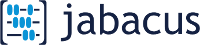version 0.0.3
February 8, 2023version 0.0.3
February 8, 2023

Location
Province:
Ss:
Sr:
Density
Snow density, γ:
kN/m3

#### Factors

Importance factor
Is:
Factors
Lower Roof, Cb:
Cw:#### Upper Roof geometry

Roof Plan dimensions
Longer dimension, l:
m
Shorter dimension, w:
m
Height of parapet, hp:
m

#### Lower Roof geometry

Roof step, h:
m
Pitch:
/12
Slippery:
Thinking...

[4.1.6.2]
S = Is[Ss(CbCwCsCa)+Sr]

#### Factors

User input values:
Ss = 1
Sr = 1
Importance Factors
ULS: Is = 1.0
SLS: Is = 0.9
Roof slope = 0 degrees
Slope Factor
For non-slippery roof:
Slope <= 30 degrees.
Cs = 1
Cb = 0.8
Density = γ = 3 kN/m3
[Structural
Commentaries
Fig G-5]

### Drift Factors & Distribution

shorter roof dimension, w = 1
longer roof dimension, l = 1 m
Characteristic roof length: lc = 2w-w2/l = 1m
Factor F = greater of:
a) F = 2
b) F = 0.35(γ*lc/Ss-6(γ*hp/Ss)2)0.5+Cb = 1.41

#### F = 2

Ca(0) = lesser of:
a) Ca(0) = (γ*h)/(CbSs)= 3.75
b) Ca(0) = F/Cb = 2.5

#### Ca(0) = 2.5

xd = lesser of:
a) xd = 5(h-CbSs/γ = 3.667 m
b) xd = 5(Ss/γ)(F-Cb) = 2 m

#### xd = 2 m

h' = h - CbCwSs/γ = 0.733m
Min dist where (Cw = 1.0) = 10*h' = 7.333 m

Cw = 1
Ca(0) = 2.5

#### 0 < x &le ( xd = 2m )

Cw = 1
Ca(x) decreases linearly over 0 < x &le xd
Ca(x) = 2.5 - 0.75 * x (x in meters)

Cw = 1
Ca(xd) = 1

Cw = 1.0
Ca(x) = 1

#### SULS = 1.0[1(0.8*1.0*1*1)+1] = 1.8 kPa

##### SSLS = 0.9[1(0.8*1.0*1*1)+1] = 1.62 kPa

By using Jabacus or information derived from this Service, you have agreed to the Terms of Service.

This information must be read or used in conjunction with Part 4 and the Structural Commentaries of the NBCC 2005. In the event of discrepancies, Part 4 and the Structural Commentaries of the NBCC 2005 shall govern.